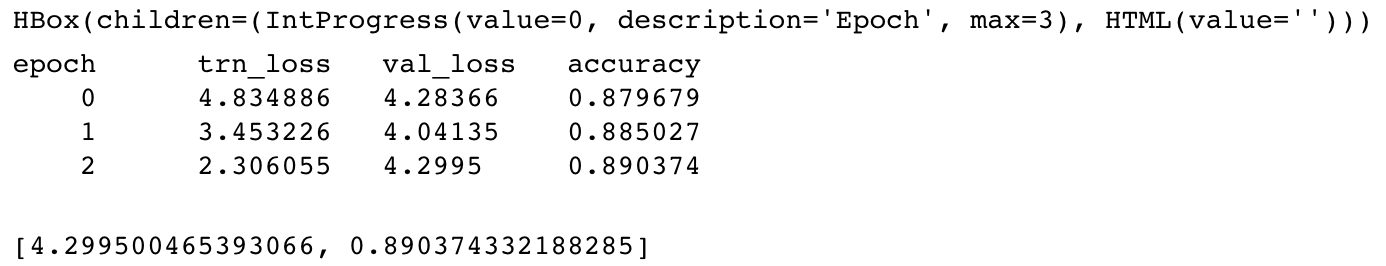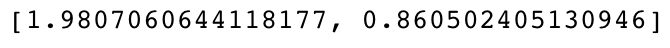# Reproducing SOTA Commonsense Reasoning Result in fast.ai with a OpenAI’s Pretrained Transformer Language Model

Published:

I wanted to write this blog post to share a bit of interesting code I’ve been working on recently. Earlier this year, OpenAI achieved SOTA results on a diverse set of NLP tasks and datasets utilizing unsupervised pretraining, nearly identically the same approach as the one ULMFiT used to achieve SOTA on several text classification datasets. However, OpenAI used the new Transformer architecture instead of the AWD LSTM used by ULMFiT and trained on a billion token corpus instead of ULMFiT’s Wikitext-103.

I’ve been exploring the Transformer architecture for various applications and datasets for the last couple months. Originally, I was examining language modeling on Wikitext-2 with the fast.ai framework and Transformer architecture, which I posted about on the fast.ai forums. Unfortunately, that was an erroneous result. I’ve been fixing various bugs and am still working on training a WT103 Transformer LM and have been looking at moving the models in Facebook’s fairseq pytorch toolkit over to fast.ai in some form. I’m also very excited to attempt this in the brand new fastai v1.0 release.

While pursuing this work, I came across a PyTorch port of OpenAI’s Transformer code that is able to load OpenAI’s pretrained weights. So, as an exercise, I thought it would be interesting to see if I could use their code and the fast.ai framework to reproduce OpenAI’s result on the ROCStories commonsense reasoning dataset. I’m excited by the possibility of including some pretrained Transformer models (one on WT103 and/or one that uses OpenAI’s version) in fast.ai v1.0 so that others can take advantage of a model that might be superior to the AWD LSTM used in ULMFiT.

Once I did this, I additionally tried to do a quick-and-dirty blending of this code and the ULMFiT IMDB code to see if I could get similar or better results with OpenAI’s Transformer. Unfortunately, I didn’t get good results, and I have several ideas as to what the problem might be. Unfortunately, I cannot pursue this specific problem any further at this time, so I have pushed my work to a Github repo so that anyone interested can dig in and try to improve on this themselves. I will attempt to give a brief overview of different pieces of the code here for the benefit of anyone wanting to work with this code.

## Reproducing ROCStories Result

The code can be found in the ROC_0 notebook.

• Text Encoding (BPE)
• I copied the PyTorch code directly for dealing with text encoding and used their vocabulary files from the pretrained model.
• Dataset
• All of the code in this section is copied directly from the PyTorch port as well.
class RocDataset(Dataset):
def __init__(self, x, y):
self.x,self.y=x,y

def __getitem__(self, idx):
x = self.x[idx]
return np.array(x),self.y[idx]

def __len__(self): return len(self.x)

trn_ds = RocDataset(trX, trY)
val_ds = RocDataset(vaX, vaY)
tst_ds = RocDataset(teX, teY)
trn_samp = SortishSampler(trX, key=lambda x: len(trX[x]), bs=8)
val_samp = SortSampler(vaX, key=lambda x: len(vaX[x]))
tst_samp = SortSampler(teX, key=lambda x: len(teX[x]))
PATH = Path('data')
md = ModelData(PATH, trn_dl, val_dl, tst_dl)

• This is how I put the data from the OpenAI data functions into fast.ai form. I used Sortish Sampler for the training dataset to group the sentences by sequence length, then used a DataLoader instance for the train, validation, and test sets.
• Model
• I copied the PyTorch code for the actual Transformer nn.Module implementation directly, the code to add task-specific output heads, and the code to load their pretrained weights.
• Optimization
• I used the “OpenAIAdam” implementation of AdamW from the PyTorch port. Ideally, I’d like to see fast.ai’s implementation of AdamW used because OpenAI’s optimizer takes care of linear warm up and decay internally (you initialize it with the total number of iterations). However, I didn’t have the time to both translating the minute optimization details into fast.ai and this reproduced the result fine.
• Loss
• OpenAI uses an auxiliary loss function with two output heads (one for language modeling, and one for the task-specific output) instead of discriminative learning rates like ULMFiT to prevent catastrophic forgetting of pretrained weights. So, I wrote my own loss function to use as my learner’s criterion to try and match the way OpenAI did it:
def MCLoss(lm_logits, clf_logits, X, Y, lm_coef=0.5):
x_shifted = X[:, :, 1:, 0].contiguous().view(-1)  # Shape: 252
"""M = torch.ne(X[:, :, :, 0] , T(torch.zeros(X.size()[:-1], dtype=torch.long))).to(torch.float)
M = M.view(-1, M.size(2))
lm_losses = F.cross_entropy(lm_logits, x_shifted, reduction='none')
lm_losses = lm_losses.view(X.size(0) * X.size(1), X.size(2) - 1)
lm_losses = lm_losses * M[:, 1:]
lm_losses = lm_losses.sum(1) / torch.sum(M[:, 1:], 1)

clf_losses = F.cross_entropy(clf_logits, Y, reduction='none')"""

lm_losses = F.cross_entropy(lm_logits, x_shifted, ignore_index=0, reduction='elementwise_mean')
clf_losses = F.cross_entropy(clf_logits, Y, reduction='sum')

train_loss = clf_losses + lm_coef * lm_losses
return train_loss

learn.crit = MCLoss

• Originally, I tried to mimic the way that they use a mask to ignore padded parts of the variable by rewriting the stepper to pass it along, but it was complicated and didn’t work. Instead, I was able to reproduce their results by telling F.cross_entropy to ignore 0 (the index for padding), and using different arguments for “reduction” to mimic the way that they summed and averaged the two losses in the original code.
• Training

learn.metrics = [accuracy]
learn.fit(6.25e-5, 3, cycle_len=1, stepper=RocStepper)• I called learn.fit with the learning rate parameter from OpenAI, but did not bother to use fast.ai’s learning rate scheduling because the OpenAIAdam class takes care of that internally. Once again, I’d ideally like to see if this result could be reproduced fully utilizing fast.ai’s training API.
• Results

from fastai.metrics import accuracy
def my_test(model, dl):
model.eval()
batch_cnts,loss,res = [], [], []
t = tqdm(iter(dl), leave=False, total=len(dl), miniters=0)
for inputs, targets in t:
lm_logits, clf_logits = model(inputs)
l = MCLoss(lm_logits, clf_logits, inputs, targets)
batch_cnts.append(inputs.size(0))
loss.append(to_np(l))
res.append([accuracy(datafy(clf_logits), datafy(targets))])
return [np.average(loss, 0, weights=batch_cnts)] + list(np.average(np.stack(res), 0, weights=batch_cnts))

my_test(learn.model, tst_dl)• In this version of the notebook, I got 86.05% accuracy on the test set, which is above the OpenAI’s median result of 85.8% but worse than their best reported result of 86.5%. I once surpassed their maximum accuracy, and in other runs I have gotten results in the 84-86% range.

## Attempting to Apply to IMDB classification

The code can be found in the openai_imdb notebook. I’m pretty sure my abysmal results on IMDB have to do with data processing stuff I don’t have the time to dig into. Either the preprocessing/tokenization of the raw text, or (more likely) the fact that I had to cut off the number of tokens from each review at 256 with a max_len variable (memory constraints). OpenAI’s transformer has been trained on sequences of up to 512 tokens, but I couldn’t use the maximum 512 without running into memory problems. Additionally, I believe that some of the IMDB reviews are even longer than 512 words. I have provided this messy and erroneous code just in case anyone is interested in seeing if they want to use my work to make a direct comparison to ULMFiT somehow.

Tags: